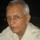Coffee Room
Discuss anything here - everything that you wish to discuss with fellow engineers.
12773 Members
Join this group to post and comment.Carlos Rubiano • May 28, 2016

Not sure I understand this about Young's Modulus

I am reading the book "Structures or why things don't fall down" by J.E. Gordon, and he states that "Young's modulus may be regarded as that stress which would double the length of the material (i.e. the stress at 100 per cent strain) - if the material did not break first - the numbers involved are often large".

The sentence is a little oddly worded, but is it saying that the value of the Young's modulus for some material represents the amount of stress required to double the length of that material?David Rubiano
is it saying that the value of the Young's modulus for some material represents the amount of stress required to double the length of that material?
That is correct.
Tensile Modulus is defined as the
"ratio of stress (force per unit area) along an axis to strain (ratio of deformation over initial length) along that axis"

Let us say that for some material for a given stress 's' the material increases the length by 0.01(1%). The Elastic modulus is s/0.01 = 100 s, which represents the stress to double the length. However, no real material can uniformly deform to actually double its initial unstressed length.Ankush Sharma • Jun 2, 2016
David Rubiano
but is it saying that the value of the Young's modulus for some material represents the amount of stress required to double the length of that material?
Yes exactly, but just one correction "not some materials but all the materials".
As, elastic modulus is the ratio of stress to strain, means the amount of stress required to produce unit strain.
Now what is the meaning of unit strain?
Means strain=1, and when it will become 1?-- when the change in length is equal to original length, which directly means the length of material doubles up.